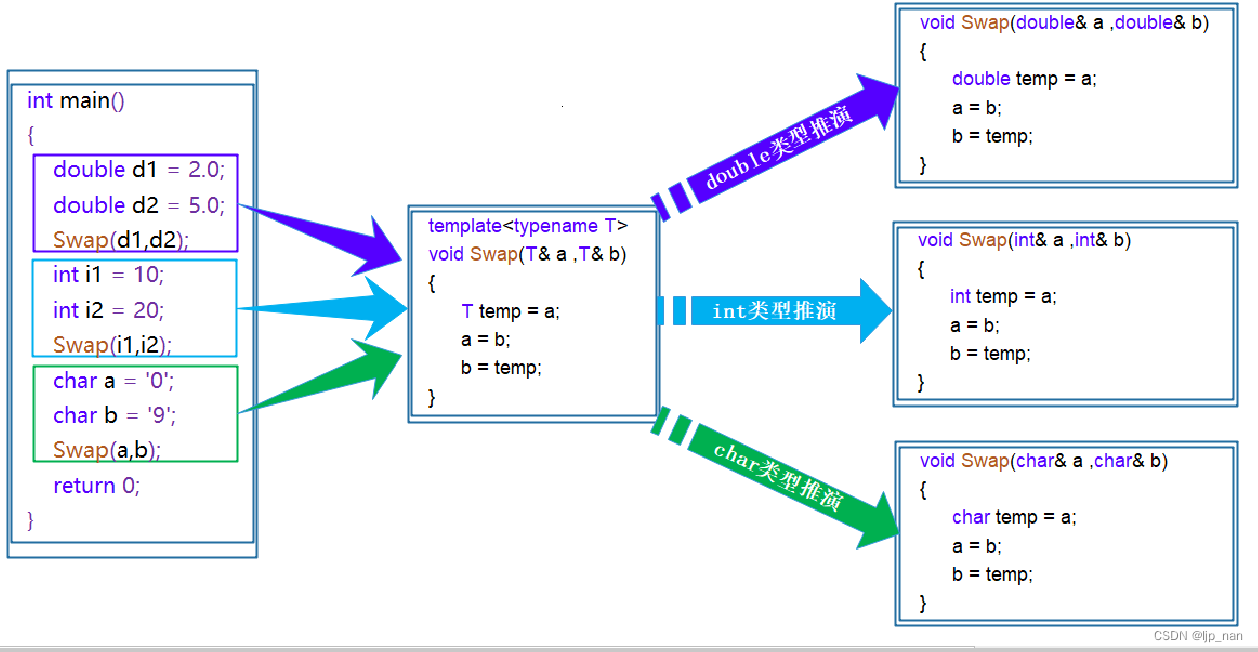## C++ ------ 模板初阶

chatgpt/2023/9/24 1:54:11

• 泛型编程
• 模板
• 函数模板
• 概念
• 原理
• 函数模板的实例化
• 类模板

# 泛型编程

``````void Swap(int& left, int& right)
{int temp = left;left = right;right = temp;
}
void Swap(double& left, double& right)
{double temp = left;left = right;right = temp;
}
void Swap(char& left, char& right)
{char temp = left;left = right;right= temp;
}
``````

# 模板

## 函数模板

``````template <typename T>
void Swap(T& a, T& b)
{T temp = a;a = b;b = temp;
}
``````

## 原理## 函数模板的实例化

``````template <typename T>T Add(const T& left, const T& right)
{return left + right;
}int  main()
}
``````

``````//通用加法函数
//这样写就不会发生两个类型不同，编译器不知道实例化成哪个的问题了
//但是这样会使精确度下降
template <typename T1,typename T2>
{return left + right;
}int main()
{int a = 1;double b = 1.1;cout << Add(a, b) << endl;
}
``````

## 类模板

``````template<class T1,class T2,……，class Tn>
class 类模板名
{//成员函数
}
``````

``````template <typename T1>
//模板类
class Stack
{
public:Stack(int capacity = 3){_array = new T1[capacity];_capacity = capacity;_top = 0;}void CheckCapacity(){if (_top == _capacity){T1* temp = (T1*)realloc(_array, sizeof(T1) * _capacity * 2);if(NULL == temp){perror("realloc failed!\n");return;}_array = temp;_capacity *= 2;cout << "扩容成功!" << endl;}}void PushStack(T1 x){CheckCapacity();_array[_top] = x;_top++;}void PopStack(){if (_top > 0){_top--;}else{cout << "退栈失败，栈已为空！" << endl;return;}}bool EmptyStack(){return _top == 0;}T1 StackTop(){return _array[_top - 1];}~Stack(){delete[] _array;_array = NULL;_capacity = _top = 0;}
private:T1* _array;int _capacity;int _top;
};
int main()
{Stack<int> s1;s1.PushStack(1);s1.PushStack(2);s1.PushStack(3);s1.PushStack(4);s1.PushStack(5);s1.PushStack(6);s1.PushStack(7);while (!s1.EmptyStack()){cout << s1.StackTop() << endl;s1.PopStack();}/*Stack<double> s2;Stack<short> s3;*/return 0;
}
``````

`Stack<T> :: Stack() //在类外定义构造函数`